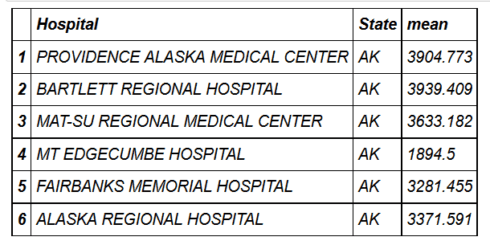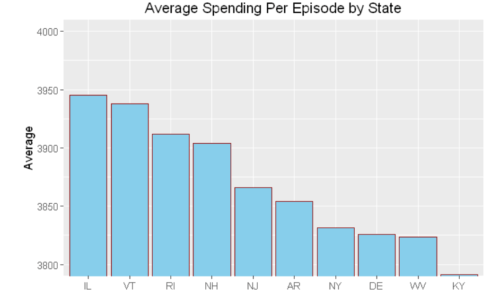Want to share your content on R-bloggers? click here if you have a blog, or here if you don't.

There are 2 packages that make data manipulation in R fun. These are `dplyr` and `data.table`. Both packages have their strengths. While `dplyr` is more elegant and resembles natural language, `data.table` is succinct and we can do a lot with `data.table` in just a single line. Further, `data.table` is, in some cases, faster (see benchmark here) and it may be a go-to package when performance and memory are constraints. You can read comparison of `dplyr` and `data.table` from Stack Overflow and Quora.

You can get reference manual and vignettes for `data.table` here and for `dplyr` here. You can read other tutorial about `dplyr` published at DataScience+

## Background

I am a long time `dplyr` and `data.table` user for my data manipulation tasks. For someone who knows one of these packages, I thought it could help to show codes that perform the same tasks in both packages to help them quickly study the other. If you know either package and have interest to study the other, this post is for you.

### dplyr

dplyr has 5 verbs which make up the majority of the data manipulation tasks we perform. Select: used to select one or more columns; Filter: used to select some rows based on specific criteria; Arrange: used to sort data based on one or more columns in ascending or descending order; Mutate: used to add new columns to our data; Summarise: used to create chunks from our data.

### data.table

data.table has a very succinct general format: DT[i, j, by], which is interpreted as: Take DT, subset rows using i, then calculate j grouped by by.

## Data manipulation

First we will install some packages for our project.

```library(dplyr)
library(data.table)
library(lubridate)
library(jsonlite)
library(tidyr)
library(ggplot2)
library(compare)
```

The data we will use here is from DATA.GOV. It is Medicare Hospital Spending by Claim and it can be downloaded from here. Let’s download the data in JSON format using the `fromJSON` function from the jsonlite package. Since JSON is a very common data format used for asynchronous browser/server communication, it is good if you understand the lines of code below used to get the data. You can get an introductory tutorial on how to use the jsonlite package to work with JSON data here and here. However, if you want to focus only on the `data.table` and `dplyr` commands, you can safely just run the codes in the two cells below and ignore the details.

```spending=fromJSON("https://data.medicare.gov/api/views/nrth-mfg3/rows.json?accessType=DOWNLOAD")
names(spending)
"meta" "data"

meta=spending\$meta
hospital_spending=data.frame(spending\$data)
colnames(hospital_spending)=make.names(meta\$view\$columns\$name)
hospital_spending=select(hospital_spending,-c(sid:meta))

glimpse(hospital_spending)
Observations: 70598
Variables:
\$ Hospital.Name                       (fctr) SOUTHEAST ALABAMA MEDICAL CENT...
\$ Provider.Number.                    (fctr) 010001, 010001, 010001, 010001...
\$ State                               (fctr) AL, AL, AL, AL, AL, AL, AL, AL...
\$ Period                              (fctr) 1 to 3 days Prior to Index Hos...
\$ Claim.Type                          (fctr) Home Health Agency, Hospice, I...
\$ Avg.Spending.Per.Episode..Hospital. (fctr) 12, 1, 6, 160, 1, 6, 462, 0, 0...
\$ Avg.Spending.Per.Episode..State.    (fctr) 14, 1, 6, 85, 2, 9, 492, 0, 0,...
\$ Avg.Spending.Per.Episode..Nation.   (fctr) 13, 1, 5, 117, 2, 9, 532, 0, 0...
\$ Percent.of.Spending..Hospital.      (fctr) 0.06, 0.01, 0.03, 0.84, 0.01, ...
\$ Percent.of.Spending..State.         (fctr) 0.07, 0.01, 0.03, 0.46, 0.01, ...
\$ Percent.of.Spending..Nation.        (fctr) 0.07, 0.00, 0.03, 0.58, 0.01, ...
\$ Measure.Start.Date                  (fctr) 2014-01-01T00:00:00, 2014-01-0...
\$ Measure.End.Date                    (fctr) 2014-12-31T00:00:00, 2014-12-3...```

As shown above, all columns are imported as factors and let’s change the columns that contain numeric values to numeric.

```cols = 6:11; # These are the columns to be changed to numeric.
hospital_spending[,cols] <- lapply(hospital_spending[,cols], as.numeric)```

The last two columns are measure start date and measure end date. So, let’s use the `lubridate` package to correct the classes of these columns.

```cols = 12:13; # These are the columns to be changed to dates.
hospital_spending[,cols] <- lapply(hospital_spending[,cols], ymd_hms)```

Now, let’s check if the columns have the classes we want.

```sapply(hospital_spending, class)
\$Hospital.Name
"factor"
\$Provider.Number.
"factor"
\$State
"factor"
\$Period
"factor"
\$Claim.Type
"factor"
\$Avg.Spending.Per.Episode..Hospital.
"numeric"
\$Avg.Spending.Per.Episode..State.
"numeric"
\$Avg.Spending.Per.Episode..Nation.
"numeric"
\$Percent.of.Spending..Hospital.
"numeric"
\$Percent.of.Spending..State.
"numeric"
\$Percent.of.Spending..Nation.
"numeric"
\$Measure.Start.Date
"POSIXct" "POSIXt"
\$Measure.End.Date
"POSIXct" "POSIXt"
```

## Create data table

We can create a data.table using the `data.table()` function.

```hospital_spending_DT = data.table(hospital_spending)
class(hospital_spending_DT)
"data.table" "data.frame" ```

## Select certain columns of data

To select columns, we use the verb `select` in `dplyr`. In `data.table`, on the other hand, we can specify the column names.

### Selecting one variable

Let’s selet the “Hospital Name” variable

```from_dplyr = select(hospital_spending, Hospital.Name)
from_data_table = hospital_spending_DT[,.(Hospital.Name)]```

Now, let’s compare if the results from `dplyr` and `data.table` are the same.

```compare(from_dplyr,from_data_table, allowAll=TRUE)
TRUE
dropped attributes```

### Removing one variable

```from_dplyr = select(hospital_spending, -Hospital.Name)
from_data_table = hospital_spending_DT[,!c("Hospital.Name"),with=FALSE]
compare(from_dplyr,from_data_table, allowAll=TRUE)
TRUE
dropped attributes```

we can also use `:=` function which modifies the input `data.table` by reference.
We will use the `copy() `function, which deep copies the input object and therefore any subsequent update by reference operations performed on the copied object will not affect the original object.

```DT=copy(hospital_spending_DT)
DT=DT[,Hospital.Name:=NULL]
"Hospital.Name"%in%names(DT)FALSE ```

We can also remove many variables at once similarly:

```DT=copy(hospital_spending_DT)
DT=DT[,c("Hospital.Name","State","Measure.Start.Date","Measure.End.Date"):=NULL]
c("Hospital.Name","State","Measure.Start.Date","Measure.End.Date")%in%names(DT)
FALSE FALSE FALSE FALSE ```

### Selecting multiple variables

Let’s select the variables:
Hospital.Name,State,Measure.Start.Date,and Measure.End.Date.

```from_dplyr = select(hospital_spending, Hospital.Name,State,Measure.Start.Date,Measure.End.Date)
from_data_table = hospital_spending_DT[,.(Hospital.Name,State,Measure.Start.Date,Measure.End.Date)]
compare(from_dplyr,from_data_table, allowAll=TRUE)
TRUE
dropped attributes```

### Dropping multiple variables

Now, let’s remove the variables Hospital.Name,State,Measure.Start.Date,and Measure.End.Date from the original data frame hospital_spending and the data.table hospital_spending_DT.

```from_dplyr = select(hospital_spending, -c(Hospital.Name,State,Measure.Start.Date,Measure.End.Date))
from_data_table = hospital_spending_DT[,!c("Hospital.Name","State","Measure.Start.Date","Measure.End.Date"),with=FALSE]
compare(from_dplyr,from_data_table, allowAll=TRUE)
TRUE
dropped attributes```

`dplyr` has functions `contains()`, `starts_with()` and, `ends_with()` which we can use with the verb select. In `data.table`, we can use regular expressions. Let’s select columns that contain the word Date to demonstrate by example.

```from_dplyr = select(hospital_spending,contains("Date"))
from_data_table = subset(hospital_spending_DT,select=grep("Date",names(hospital_spending_DT)))
compare(from_dplyr,from_data_table, allowAll=TRUE)
TRUE
dropped attributes

names(from_dplyr)
"Measure.Start.Date" "Measure.End.Date" ```

## Rename columns

```setnames(hospital_spending_DT,c("Hospital.Name", "Measure.Start.Date","Measure.End.Date"), c("Hospital","Start_Date","End_Date"))
names(hospital_spending_DT)
"Hospital" "Provider.Number." "State" "Period" "Claim.Type" "Avg.Spending.Per.Episode..Hospital." "Avg.Spending.Per.Episode..State." "Avg.Spending.Per.Episode..Nation." "Percent.of.Spending..Hospital." "Percent.of.Spending..State." "Percent.of.Spending..Nation." "Start_Date" "End_Date"

hospital_spending = rename(hospital_spending,Hospital= Hospital.Name, Start_Date=Measure.Start.Date,End_Date=Measure.End.Date)
compare(hospital_spending,hospital_spending_DT, allowAll=TRUE)
TRUE
dropped attributes```

## Filtering data to select certain rows

To filter data to select specific rows, we use the verb `filter` from `dplyr` with logical statements that could include regular expressions. In `data.table`, we need the logical statements only.

### Filter based on one variable

```from_dplyr = filter(hospital_spending,State=='CA') # selecting rows for California
from_data_table = hospital_spending_DT[State=='CA']
compare(from_dplyr,from_data_table, allowAll=TRUE)
TRUE
dropped attributes```

### Filter based on multiple variables

```from_dplyr = filter(hospital_spending,State=='CA' & Claim.Type!="Hospice")
from_data_table = hospital_spending_DT[State=='CA' & Claim.Type!="Hospice"]
compare(from_dplyr,from_data_table, allowAll=TRUE)
TRUE
dropped attributes
from_dplyr = filter(hospital_spending,State %in% c('CA','MA',"TX"))
from_data_table = hospital_spending_DT[State %in% c('CA','MA',"TX")]
unique(from_dplyr\$State)
CA MA TX

compare(from_dplyr,from_data_table, allowAll=TRUE)
TRUE
dropped attributes```

## Order data

We use the verb `arrange` in `dplyr` to order the rows of data. We can order the rows by one or more variables. If we want descending, we have to use `desc()` as shown in the examples.The examples are self-explanatory on how to sort in ascending and descending order. Let’s sort using one variable.

### Ascending

```from_dplyr = arrange(hospital_spending, State)
from_data_table = setorder(hospital_spending_DT, State)
compare(from_dplyr,from_data_table, allowAll=TRUE)
TRUE
dropped attributes```

### Descending

```from_dplyr = arrange(hospital_spending, desc(State))
from_data_table = setorder(hospital_spending_DT, -State)
compare(from_dplyr,from_data_table, allowAll=TRUE)
TRUE
dropped attributes```

### Sorting with multiple variables

Let’s sort with State in ascending order and End_Date in descending order.

```from_dplyr = arrange(hospital_spending, State,desc(End_Date))
from_data_table = setorder(hospital_spending_DT, State,-End_Date)
compare(from_dplyr,from_data_table, allowAll=TRUE)
TRUE
dropped attributes```

In `dplyr` we use the function `mutate()` to add columns. In `data.table`, we can Add/update a column by reference using `:=` in one line.

```from_dplyr = mutate(hospital_spending, diff=Avg.Spending.Per.Episode..State. - Avg.Spending.Per.Episode..Nation.)
from_data_table = copy(hospital_spending_DT)
from_data_table = from_data_table[,diff := Avg.Spending.Per.Episode..State. - Avg.Spending.Per.Episode..Nation.]
compare(from_dplyr,from_data_table, allowAll=TRUE)
TRUE
sorted
renamed rows
dropped row names
dropped attributes
from_dplyr = mutate(hospital_spending, diff1=Avg.Spending.Per.Episode..State. - Avg.Spending.Per.Episode..Nation.,diff2=End_Date-Start_Date)
from_data_table = copy(hospital_spending_DT)
from_data_table = from_data_table[,c("diff1","diff2") := list(Avg.Spending.Per.Episode..State. - Avg.Spending.Per.Episode..Nation.,diff2=End_Date-Start_Date)]
compare(from_dplyr,from_data_table, allowAll=TRUE)
TRUE
dropped attributes```

## Summarizing columns

We can use the `summarize()` function from` dplyr `to create summary statistics.

```summarize(hospital_spending,mean=mean(Avg.Spending.Per.Episode..Nation.))
mean 8.772727

hospital_spending_DT[,.(mean=mean(Avg.Spending.Per.Episode..Nation.))]
mean 8.772727

summarize(hospital_spending,mean=mean(Avg.Spending.Per.Episode..Nation.),
maximum=max(Avg.Spending.Per.Episode..Nation.),
minimum=min(Avg.Spending.Per.Episode..Nation.),
median=median(Avg.Spending.Per.Episode..Nation.))
mean  maximum  minimum  median
8.77  19       1        8.5

hospital_spending_DT[,.(mean=mean(Avg.Spending.Per.Episode..Nation.),
maximum=max(Avg.Spending.Per.Episode..Nation.),
minimum=min(Avg.Spending.Per.Episode..Nation.),
median=median(Avg.Spending.Per.Episode..Nation.))]
mean  maximum  minimum  median
8.77  19       1        8.5```

We can calculate our summary statistics for some chunks separately. We use the function `group_by()` in `dplyr` and in `data.table`, we simply provide `by`.

`head(hospital_spending_DT[,.(mean=mean(Avg.Spending.Per.Episode..Hospital.)),by=.(Hospital)])````mygroup= group_by(hospital_spending,Hospital)
from_dplyr = summarize(mygroup,mean=mean(Avg.Spending.Per.Episode..Hospital.))
from_data_table=hospital_spending_DT[,.(mean=mean(Avg.Spending.Per.Episode..Hospital.)), by=.(Hospital)]
compare(from_dplyr,from_data_table, allowAll=TRUE)

TRUE
sorted
renamed rows
dropped row names
dropped attributes```

We can also provide more than one grouping condition.

```head(hospital_spending_DT[,.(mean=mean(Avg.Spending.Per.Episode..Hospital.)),
by=.(Hospital,State)])``````mygroup= group_by(hospital_spending,Hospital,State)
from_dplyr = summarize(mygroup,mean=mean(Avg.Spending.Per.Episode..Hospital.))
from_data_table=hospital_spending_DT[,.(mean=mean(Avg.Spending.Per.Episode..Hospital.)), by=.(Hospital,State)]
compare(from_dplyr,from_data_table, allowAll=TRUE)
TRUE
sorted
renamed rows
dropped row names
dropped attributes```

## Chaining

With both `dplyr` and `data.table`, we can chain functions in succession. In `dplyr`, we use pipes from the `magrittr` package with `%>%` which is really cool. `%>% `takes the output from one function and feeds it to the first argument of the next function. In `data.table`, we can use `%>%` or `[` for chaining.

```from_dplyr=hospital_spending%>%group_by(Hospital,State)%>%summarize(mean=mean(Avg.Spending.Per.Episode..Hospital.))
from_data_table=hospital_spending_DT[,.(mean=mean(Avg.Spending.Per.Episode..Hospital.)), by=.(Hospital,State)]
compare(from_dplyr,from_data_table, allowAll=TRUE)
TRUE
sorted
renamed rows
dropped row names
dropped attributes
hospital_spending%>%group_by(State)%>%summarize(mean=mean(Avg.Spending.Per.Episode..Hospital.))%>%
mutate(State = factor(State,levels = State[order(mean,decreasing =TRUE)]))%>%
ggplot(aes(x=State,y=mean))+geom_bar(stat='identity',color='darkred',fill='skyblue')+
xlab("")+ggtitle('Average Spending Per Episode by State')+
ylab('Average')+ coord_cartesian(ylim = c(3800, 4000))``````hospital_spending_DT[,.(mean=mean(Avg.Spending.Per.Episode..Hospital.)),
by=.(State)][order(-mean)][1:10]%>%
mutate(State = factor(State,levels = State[order(mean,decreasing =TRUE)]))%>%
ggplot(aes(x=State,y=mean))+geom_bar(stat='identity',color='darkred',fill='skyblue')+
xlab("")+ggtitle('Average Spending Per Episode by State')+
ylab('Average')+ coord_cartesian(ylim = c(3800, 4000))```## Summary

In this blog post, we saw how we can perform the same tasks using `data.table` and `dplyr` packages. Both packages have their strengths. While `dplyr` is more elegant and resembles natural language, `data.table` is succinct and we can do a lot with `data.table `in just a single line. Further, `data.table` is, in some cases, faster and it may be a go-to package when performance and memory are the constraints.

You can get the code for this blog post at my GitHub account.

This is enough for this post. If you have any questions or feedback, feel free to leave a comment.

Related Post